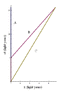granpa
suppose we have a stationary observer 'A' at the origin. at t=0 rockets 'B' and 'C' pass the origin moving at gamma=10 but rocket B stops. when rocket 'C' reaches some point along the x-axis rocket 'B' accelerates to gamma=10 as measured by rocket 'C'. when rockets 'B' and 'C' meet its over. who ages more, rocket 'B' or 'C'? let us also assume that there exists a long line of evenly spaced clocks, perfectly synchronized to observer 'A', along the x axis.

obviously if rocket 'C' is considered to be stationary then we just have the twins paradox. but what is the result if we look at it from a different point of view? obviously the result should be the same.

Mentor
suppose we have a stationary observer 'A' at the origin. at t=0 rockets 'B' and 'C' pass the origin moving at gamma=10 but rocket B stops. when rocket 'C' reaches some point along the x-axis rocket 'B' accelerates to gamma=10 as measured by rocket 'C'. when rockets 'B' and 'C' meet its over. who ages more, rocket 'B' or 'C'? let us also assume that there exists a long line of evenly spaced clocks, perfectly synchronized to observer 'A', along the x axis.

obviously if rocket 'C' is considered to be stationary then we just have the twins paradox. but what is the result if we look at it from a different point of view? obviously the result should be the same.
As always I would recommend the spacetime geometric approach. Here is an example, I used v = .6 c (gamma = 1.25) so that plotting it is easier and picked the inertial reference frame where A is at rest. Units of time are years and units of distance are lightyears so that c = 1.

Recall that the four-vector is (ct,x) and that the spacetime interval along a four-vector dr = (c dt,dx) is ds² = c²dt²-dx² = dr.dr and that ds/c is the proper time experienced by a clock moving along dr.

a0 = b0 = c0 = (0,0)
a1 = b1 = (5,0)
c1 = (5,3)
a2 = (15.625,0)
b2 = c2 = (15.625,9.375)

Let da1=a1-a0 and da2 = a2-a1 then total accumulated time on clock A is:
sqrt(da1.da1)/c + sqrt(da2.da2)/c = sqrt(5²-0²) + sqrt(10.625²-0²) = 15.625

Let db1=b1-b0 and db2 = b2-b1 then total accumulated time on clock B is:
sqrt(db1.db1)/c + sqrt(db2.db2)/c = sqrt(5²-0²) + sqrt(10.625²-9.375²) = 10

Let dc1=c1-c0 and dc2 = c2-c1 then total accumulated time on clock C is:
sqrt(dc1.dc1)/c + sqrt(dc2.dc2)/c = sqrt(5²-3²) + sqrt(10.625²-6.375²) = 12.5

I will leave it as an exercise for you to show that the intervals are the same in the inertial frame where C is at rest.

#### Attachments

•twins.GIF
5.1 KB · Views: 412